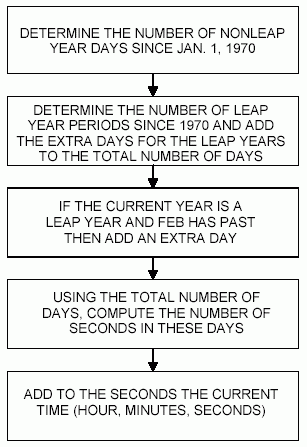# DS1371/DS1372/DS1374 32-Bit Binary Counter Time Conversion

### Abstract

This application note addresses how to convert the 32-bit time value into a date and time value that can be put in the form of MM/DD/YYYY, HH:MM:SS. An algorithm for converting from a date and time to binary seconds is also described.

### Introduction

The DS1371 and DS1374 have a 32-bit binary counter RTC with a 2-wire interface. The 32-bit binary counters are designed to continuously count time in seconds. This application note addresses how to convert the 32-bit time value into a date and time value that can be put in the form of MM/DD/YYYY, HH:MM:SS. An algorithm for converting from a date and time to binary seconds is also described.

Both devices accumulate time information in a 4-byte register as the number of seconds since some arbitrary reference date. The date used in this application note is the same used with the UNIX operating system, the reference date January 1, 1970, often referred to as Unix Epoch. Note: Because of the reference date used and the use of a 32-bit counter, this algorithm rolls over on Tuesday, January 19, 03:14:07, 2038.

### Binary Seconds to Date/Time

Figure 1 shows the basic algorithm used to convert raw seconds to a date/time. Below is a C implementation of the algorithm.

```// AppNote517.cpp : Defines the entry point for the console application.
//

#include "stdafx.h"

// this structure is just the local version of Visual C tm structure in time.h
struct tm_struct {
int tm_sec;     /* seconds after the minute - [0,59]    */
int tm_min;     /* minutes after the hour - [0,59]      */
int tm_hour;    /* hours since midnight - [0,23]        */
int tm_mday;    /* day of the month - [1,31]            */
int tm_mon;     /* months since January - [0,11]        */
int tm_year;    /* years since 1900                     */
int tm_wday;    /* days since Sunday - [0,6]            */
int tm_yday;    /* days since January 1 - [0,365]       */
int tm_isdst;   /* daylight savings time flag           */  // NOT USED
};

// this array represents the number of days in one non-leap year at
//    the beginning of each month
unsigned long DaysToMonth = {
0,31,59,90,120,151,181,212,243,273,304,334,365
};

unsigned long DS1371_DateToBinary(tm_struct *datetime) {

unsigned long iday;
unsigned long val;

iday = 365 * (datetime->tm_year - 70) + DaysToMonth[datetime->tm_mon-1] + (datetime->tm_mday - 1);
iday = iday + (datetime->tm_year - 69) / 4;
if ((datetime->tm_mon > 2) && ((datetime->tm_year % 4) == 0)) {
iday++;
}
val = datetime->tm_sec + 60 * datetime->tm_min + 3600 * (datetime->tm_hour + 24 * iday);
return val;
}

void DS1371_BinaryToDate(unsigned long binary,tm_struct *datetime) {

unsigned long hour;
unsigned long day;
unsigned long minute;
unsigned long second;
unsigned long month;
unsigned long year;

unsigned long whole_minutes;
unsigned long whole_hours;
unsigned long whole_days;
unsigned long whole_days_since_1968;
unsigned long leap_year_periods;
unsigned long days_since_current_lyear;
unsigned long whole_years;
unsigned long days_since_first_of_year;
unsigned long days_to_month;
unsigned long day_of_week;

whole_minutes = binary / 60;
second = binary - (60 * whole_minutes);                 // leftover seconds

whole_hours  = whole_minutes / 60;
minute = whole_minutes - (60 * whole_hours);            // leftover minutes

whole_days   = whole_hours / 24;
hour         = whole_hours - (24 * whole_days);         // leftover hours

whole_days_since_1968 = whole_days + 365 + 366;
leap_year_periods = whole_days_since_1968 / ((4 * 365) + 1);

days_since_current_lyear = whole_days_since_1968 % ((4 * 365) + 1);

// if days are after a current leap year then add a leap year period
if ((days_since_current_lyear >= (31 + 29))) {
leap_year_periods++;
}
whole_years = (whole_days_since_1968 - leap_year_periods) / 365;
days_since_first_of_year = whole_days_since_1968 - (whole_years * 365) - leap_year_periods;

if ((days_since_current_lyear <= 365) && (days_since_current_lyear >= 60)) {
days_since_first_of_year++;
}
year = whole_years + 68;

// setup for a search for what month it is based on how many days have past
//   within the current year
month = 13;
days_to_month = 366;
while (days_since_first_of_year < days_to_month) {
month--;
days_to_month = DaysToMonth[month-1];
if ((month > 2) && ((year % 4) == 0)) {
days_to_month++;
}
}
day = days_since_first_of_year - days_to_month + 1;

day_of_week = (whole_days  + 4) % 7;

datetime->tm_yday =
days_since_first_of_year;      /* days since January 1 - [0,365]       */
datetime->tm_sec  = second;        /* seconds after the minute - [0,59]    */
datetime->tm_min  = minute;        /* minutes after the hour - [0,59]      */
datetime->tm_hour = hour;          /* hours since midnight - [0,23]        */
datetime->tm_mday = day;           /* day of the month - [1,31]            */
datetime->tm_wday = day_of_week;   /* days since Sunday - [0,6]            */
datetime->tm_mon  = month;         /* months since January - [0,11]        */
datetime->tm_year = year;          /* years since 1900                     */
}

int main(int argc, char* argv[])
{
tm_struct timeStruct;
unsigned long binary;

printf("DS1371_DateToBinary and DS1371_BinaryToDate example:\n\n");

timeStruct.tm_hour = 17;
timeStruct.tm_min = 32;
timeStruct.tm_sec = 53;
timeStruct.tm_year = 107;
timeStruct.tm_mday = 21;
timeStruct.tm_mon = 6;
timeStruct.tm_wday = 4;
binary = DS1371_DateToBinary(&timeStruct);
printf("DS1371_DateToBinary(&timeStruct) where timeStruct = ");
printf("%d:%d:%d, %d/%d/%d\n",timeStruct.tm_hour,timeStruct.tm_min,timeStruct.tm_sec,
timeStruct.tm_mon,timeStruct.tm_mday,timeStruct.tm_year+1900);
printf("\t\t\t\tbinary returned value = %d\n\n",binary);

DS1371_BinaryToDate(binary,&timeStruct);
printf("DS1371_BinaryToDate(binary,&timeStruct) where binary = %d\n",binary);
printf("\t\t\t\ttimeStruct -> %d:%d:%d, %d/%d/%d\n",timeStruct.tm_hour,timeStruct.tm_min,timeStruct.tm_sec,
timeStruct.tm_mon,timeStruct.tm_mday,timeStruct.tm_year+1900);

printf("\ndone.\n");

/*  Results of execution:

DS1371_DateToBinary and DS1371_BinaryToDate example:

DS1371_DateToBinary(&timeStruct) where timeStruct = 17:32:53, 6/21/2007
binary returned value = 1185039173

DS1371_BinaryToDate(binary,&timeStruct) where binary = 1185039173
timeStruct -> 17:32:53, 6/21/2007

done.

*/
return 0;
}
```Figure 1. Binary to date algorithm flow.

### Date/Time to Binary Seconds

Figure 2 shows the basic algorithm used to convert date/time to raw seconds. Below is a C implementation of the algorithm.

```unsigned long DS1371_DateToBinary(tm_struct *datetime) {

unsigned long iday;
unsigned long val;

iday = 365 * (datetime->tm_year - 70) + DaysToMonth[datetime->tm_mon]
+ (datetime->tm_mday - 1);
iday = iday + (datetime->tm_year - 69) / 4;
if ((datetime->tm_mon > 1) && ((datetime->tm_year % 4) == 0)) {
iday++;
}
val = datetime->tm_sec + 60 * datetime->tm_min + 3600
* (datetime->tm_hour + 24 * iday);
return val;}
```Figure 2. Date to binary algorithm flow.

### Testing

The C implementations used in this application note were thoroughly tested for each counter value over the 68-year span of the algorithm (from January 1, 1970 to January 18, 2038) and was found to be error free.